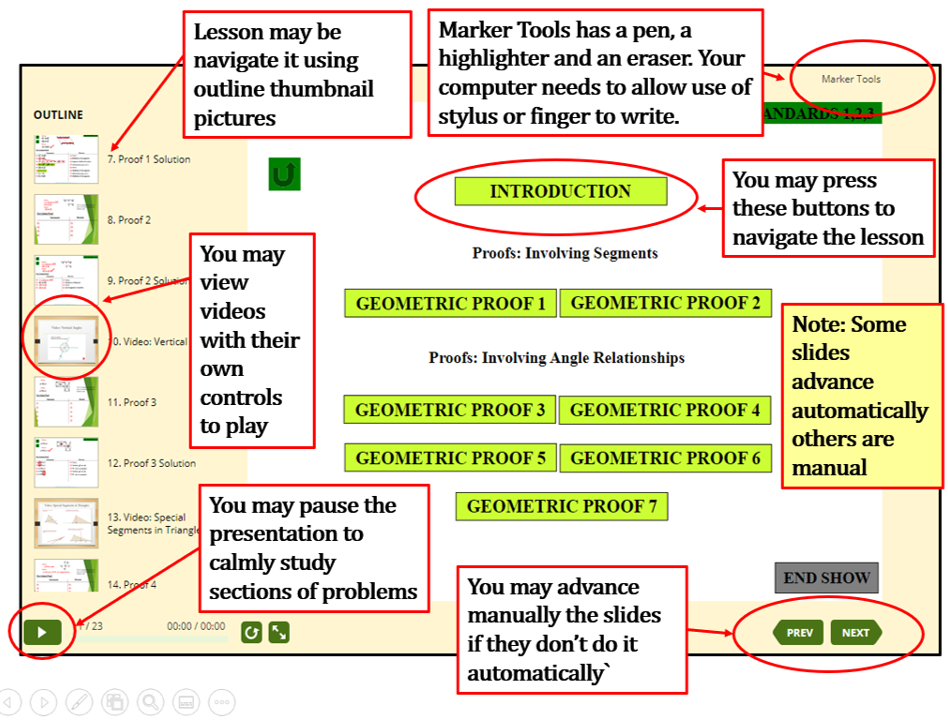# MrPerezOnlineMathTutor.com

QUICK SITE TOUR VIDEO

 Algebra 1 and 2 Geometry Basic Math Statistics and Probability Quizzes for Geometry#### The original format is in PowerPoint but have been formatted for the internet.

 Algebra 1 and 2 Geometry Basic Math Statistics and Probability Quizzes for Geometry